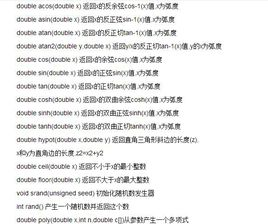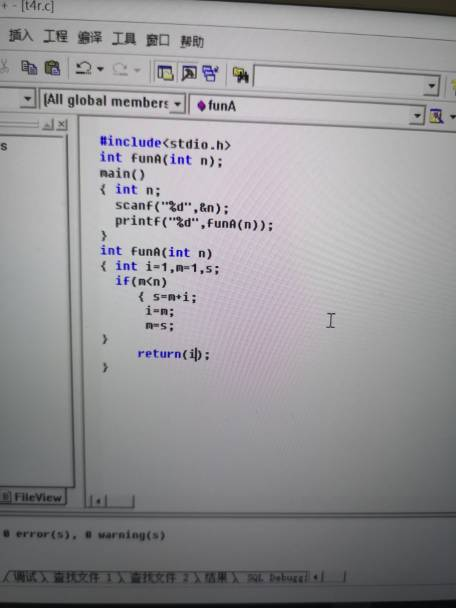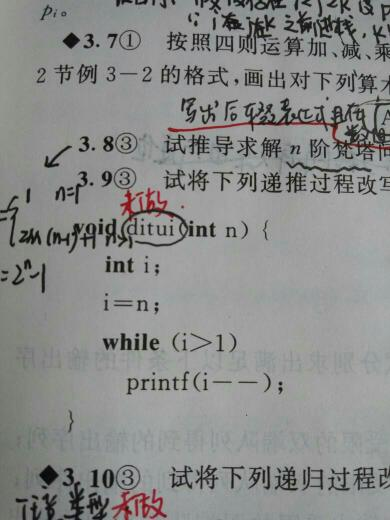# c 语言编程 函数的递归,c语言函数递归-递归,C语言入门

int

F

//递归函数

c语言函数递归C语言函数是一种函数，用来编译C语言，一般包括字符库函数，数学函数，目录函数，进程函数，诊断函数，操作函数等。

C语言中的递归函数写法

#includeint F( int n ) //递归函数

{

longsum; //if(n == 0 || n == 1) //递归终点。return1;

else

{

sum = n * F( n - 1 );

}

returnsum; //函数返回sum的值。

}

int main()

{

int n;

scanf("%d", &n); //输入n

printf("%d! = %d\n", n, F(n));

return0;

}

#includeint n; //定义全局变量n，记录输入天数int Taozi( int day ) //递归函数。

{

int num;

if( day == n )

{

return1; //递归终点。

}

else

{

num = 2 * ( Taozi( day + 1 ) + day ); //递推公式

}

return num; //返回num的值

}

int main()

{

int num; //此处num是局部变量，与递归函数中的num 意义不同scanf("%d", &n); //输入全局变量n的值

num = Taozi( 1 ); //因为是从第一天开始算，传入的参数值为 1。if(num > 1)

printf("The monkey got %d peaches in first day.\n", num);

elseprintf("The monkey got %d peach in first day.\n", num);

}

1 + 1/2 - 1/3 + 1/4 -1/5 ……

n

#include #include double plus(int x) // 这是一个返回值为浮点数的函数，写在后面的话要进行函数申明。

{

doublesum = 0;

if(x == 1) // 递归终点

{

return1;

}

else

{

if(x % 2 == 0) //当为偶数项时，符号为正sum = 1.0 / x + plus(x - 1);

else//当为奇数项时，符号为负(首项除外)sum = -1.0 / x + plus(x -1);

}

returnsum;

}

int main()

{

doublesum = 0;

int n = 0;

scanf("%d", &n);

sum = plus(n);

if(sum != 1)

printf("%06lf\n", sum);

else

printf("1\n");

return0;

}

#include #include #include voidreverse(char *str, int start, int end)

{

int t;

for(t = 0; str[t] != '\0'; t++); //计算字符串的长度//也可以 t = strlen(str);char temp; //中间变量if(end > t)

//当给的end超过字符串长度时，end直接变为t-1，end是下标所以减一

end = t - 1;

if(end <= start)

{

return; //递归终点

}

else

{

temp = str[start];

str[start] = str[end];

str[end] = temp; //交换字符reverse(str, start + 1, end - 1); //递归调用

}

return;

}

int main( )

{ charstr;

int start, end;

gets(str);

scanf("%d %d", &start, &end);

reverse( str, start, end );

printf("%s\n", str);

return0;

}

Yes或者No

#include#includeint Huiwen( char *p , int n ) // n是代表字符串长度的参数

{

if(n == 1 || n == 0)

return1;

else

{

if( *p == *(p + n - 1) ) //判断对应位置的字符是否相同

{

return Huiwen( p + 1, n - 2 );

//这里返回的是下一次调用的返回值，这样只有当所有对应字符相同时才返回 1。

}

elsereturn0;

}

}

int main()

{

char arr = {0};

int n;

gets( arr );

n = strlen( arr );

if( Huiwen( arr, n) )

{

printf("Yes\n");

}

else

{

printf("No\n");

}

return0;

}

C语言中的递归函数有什么用途？什么意义？

int f(int n){

if(n==1) return 1;

return n+f(n-1);

}

1.程序总得要有机会让它退出来，不然会变成死循环。就象这里的第一句，而且一般来说位置也通常在第一句。

2.递归还有性能开销。一是因为函数调用时参数的入栈出栈操作。二是有些问题处理不当会出现重复计算(不是很老到的程序员经常会出这样的问题，导致性能有问题，然后说递归根本没实际意义)。

c语言函数的递归调用？

Fibonacci数由小到排列后具有以下特点：前二项均为1，从第三项时，每项等于前二项的和。编写函数funA(n)，用于返回一个不小于n的最小的一个Fibonacci数。主函数如下：main(){intn;sca...

Fibonacci数由小到排列后具有以下特点：前二项均为1，从第三项时，每项等于前二项的和。编写函数funA(n)，用于返回一个不小于n的最小的一个Fibonacci数。

main()

{ int n;

scanf("%d",&n);

printf("%d",funA(n));

}int rec(int x,int n)

{

if(n==0)

return 1;

else

return rec(x,n-1)*x;

}

int main()

{

int x,n;

printf("请输入x和n\n");

scanf("%d%d",&x,&n);

printf("结果是:%d\n",rec(x,n));

}

c语言中，什么是函数的递归，能举个例子么

1 1 2 3 5 8 13 21 ........n

int fun(int n) // 这里的n代表第几个数

{

if(1 == n || 2 == n) // 第一个数

{

return 1;

}

else

{

return fun(n - 1) + fun(n - 2); // 这里就是自己调用自己，形成循环自我调用。

}

}

c语言怎么用递归调用函数的方法求n的阶乘？

1、打开VC6.0软件，新建一个C语言的项目：

2、接下来编写主程序，首先定义用来求阶乘的递归函数以及主函数。在main函数里定义变量sum求和，调用递归函数fact()，并将返回值赋予sum，最后使用printf打印sum的结果，主程序就编写完了：

3、最后运行程序，观察输出的结果。以上就是C语言使用递归求阶乘的写法：

c语言中的递归

for(int i=1; i<=n; i++)

if (i == x)

cout << 2*i; /*cout 相当于 c里面的printf,就是输出.*/

int a(int x) {

if (x = 1)

return 2; /* 第一项那肯定是2了，这个也是递归的终止条件！ */

else return a(x-1)+2; /* 函数自身调用自身是递归的一个特色 */

// zysable's tail recursive algorithm of factorial.

int fac(int x, int y) {

if (x == 1)

return y;

else return fac(x-1, y*x);}

int ff(int x) {

if (x == 0)

return 1;

else return fac(x,1);}

PS:递归不要滥用，否则程序极其无效率，要用也用尾递归。by 一名在美国的中国程序员zysable。

C语言的函数调用是递归吗？

C语言递归是什么意思

C语言中的递归是什么意思

C语言中经常说的递归是什么意思呢

#include

#include

int fac(int n)

{

if(n==0)return 1;

return n*fac(n-1);

}

void main()

{

printf("%d\n",fac(6));

}

C语言中递归函数是，非递归函数是？能否举例子？

unsigned fun(unsigned x){

if(x==1 || x==0)

return 1;

return x*fun(x-1);

}01-038250
04-25403
05-10630
11-25946
02-031293
12-136630
07-091004
03-25247
05-27233
01-221万+
07-26577
05-31337
07-251万+
12-015807
12-191023
11-141260
05-14506
10-28235
07-30128
05-18894

### “相关推荐”对你有帮助么？

•非常没帮助
•没帮助
•一般
•有帮助
•非常有帮助被折叠的  条评论 为什么被折叠?到【灌水乐园】发言点击重新获取扫码支付1.余额是钱包充值的虚拟货币，按照1:1的比例进行支付金额的抵扣。
2.余额无法直接购买下载，可以购买VIP、C币套餐、付费专栏及课程。余额充值